## Saturday, September 7, 2013

### P1 Math - Divisions (The Geek Dad Way)

In Jay Jay's communication booklet, teacher clearly stated that all students will bring back e math text books so that they can do some revision on Multiplication & Divisions.  So, I am checking on Jay Jay's concept of divisionsDad:  6 divide by 3 is how much...

Jay:  (bring up 6 fingers, and slowly cover 3) it's 2 group of 3 daddy.

Dad:  good... 18 divided by 2 is?

Jay:  (Think for a long time)...

Obviously, he has no 18 fingers.  So, he struggles....

Jay:  oh ya.  2-1-2, 2-2-4, 2-3-6, 2-4-8, 2-5-10, 2-6-12, 2-7-14, 2-8-16, 2-9-18.  It's 18.

Wow!  Amazing.  He really loves that CMA multiplication song.

But in my opinion, it could be too slow using these method.  So I decided to give him a 20 minutes lesson on division.  While driving him to the Robotics class.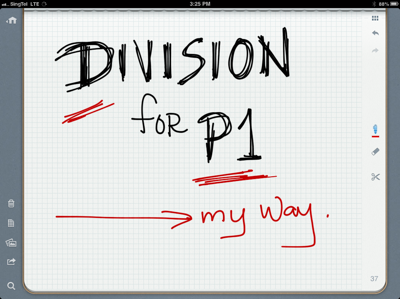So, I taught him, the first and second rule.

Rule #1:  Any number can ALWAYS be divided by the number 1 (one).  When you divide any number by 1, it will always gives that number itself.  I.e.  13 divide by 1 is 13.

Rule #2:  Any number can ALWAYS be divided by itself.  When you divide any number by itself, it always gives the number 1 (one).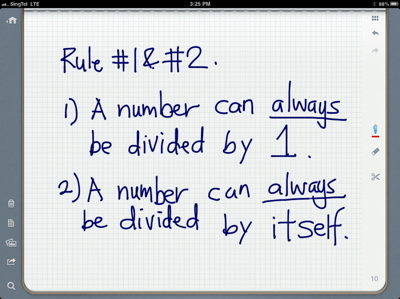Jay:  really?  (Looks a bit puzzled)

Dad:  let's try it.  1 divided by 1 equal 1.  2 divide by 1 equal 2.  3 divide 1 equal 3.  4 divide by 1 equal 4.

Jay:  oh... Really is like that daddy.

Dad:  yes, you take any number divide by 1 always gives you that SAME number.  No need to think.

Jay:  ok.

Dad:  123 divide by 1 equal to?

Jay:  123?  Very easy.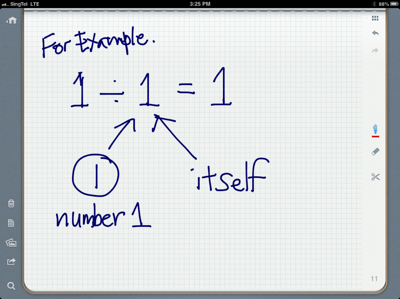I am not sure when the school going to teach this, but to me, the easiest way for Jay to learn division is to understand the math from 1-20.  And there are a few ground rules he has to learn and understand.

Rule #1 is easy to learn.  So, I have to make sure remember this always.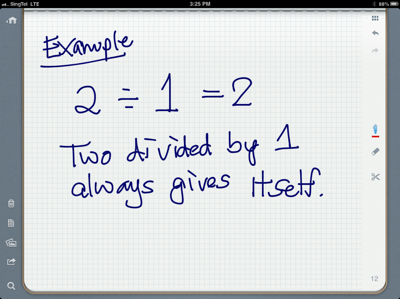Dad:  jay, now, rule #2.  Whatever number always can be divided by it self.  When that whatever number divided by itself is always equal to 1.  Very EASY.

Jay:  ok.

Dad:  1 divided by 1 is 1.  2 divided by 2 (itself) is 1.  3 divided by 3 is 1.  4 divided by 4 is 1.  165 divided by 165 is how many.

Jay:  1.

Not too bad.  Not hard to remember too.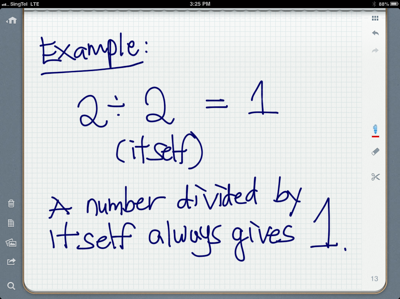I gave a lot of drills to Jay so that I make sure he understands the two basic rules.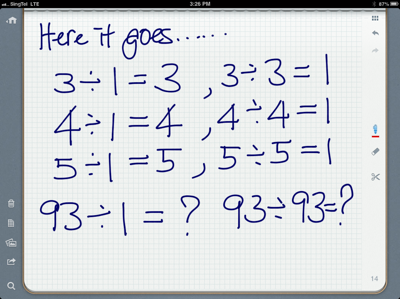Next, I taugh him that, if teacher gives you any number and as what that number can be divided by which number, without thinking, 1 and itself (that number) has to be his FIRST TWO ANSWERS.

After that, he will have to take that number slowly and in numerical order divide by the next number.

For example, 4.

Jay will have to answer 1 & 4 without thinking.

Then, he will think if 4 can be divided by 2 or not?  YES.

Lastly, he will think if 4 can be divided by 3 or not?  Which is NO.

When you ask them, you always ask if 4 to be divided by 2, then 4 has 2 groups of what number?

It is important, as this is how they been taught in class.Of course, by using groups concept, they know 4 cannot be having 3 groups of any number.

For the beginning, I think it is very important to run thru numbers 1-20 and figure out what these number can be divided by?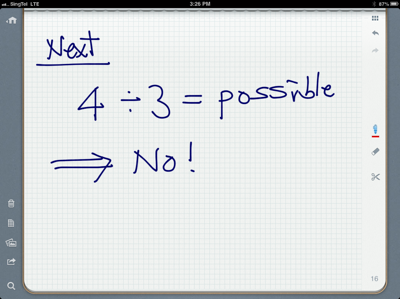So, lets move on to 5.

Then 6.

Wow, 6 not only can be divided by 1 & 6, it is divisible by 2 & 3.

Here you need to strengthen the concept of 2 groups of 3 and 3 groups of 2 IS THE SAME.

If he does not believe, ask him to count by fingers.

Jay: wow! Really is like that.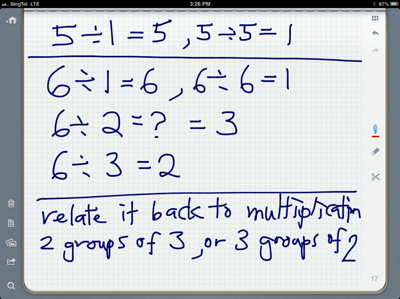Lets do 7.

And then followed by 8.

Teach them the normal method.  Start divide by 2 follow by 3,4,5,6 & 7.

A longer method.

Repetitive.

Usually kids will be bored in doing so.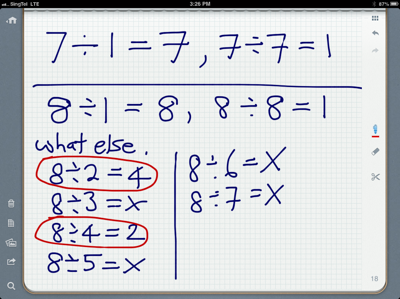Lets move on to 9 then 10.

If needed, practice another few rounds from 1 to 10.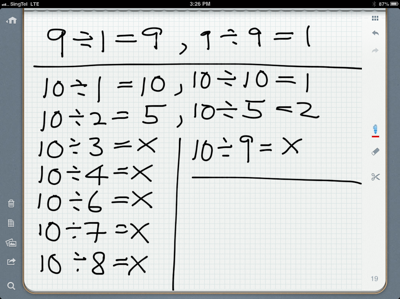Congradulations!  Jay has done division from 1-10.

List the result out on the board.  (Boogie Board)

Ask him what does he see?

Of course my Jay is not that clever be able to figure out what I want him to find.

Hahaha.

Dad:  1, 2, 3, 5 & 7 are very special numbers.

Jay:  why?

Dad:  these number can ONLY be divided by 1 or itself.  These number cannot be divided by other numbers other than 1 or itself.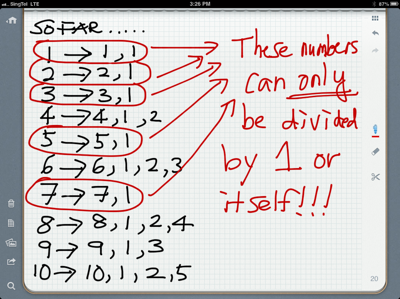These are called PRIME NUMBERS.

Any number that can ONLY ONLY ONLY ONLY be divided by itself or 1 ONLY are called PRIME NUMBERS.

Jay has been a very curious kid.  He loves to learn new things.  Remember I taught him wormhole?

As expected, he wanted to know more.

So, I play with him the next game.  Lets find the next PRIME NUMBER next to 7.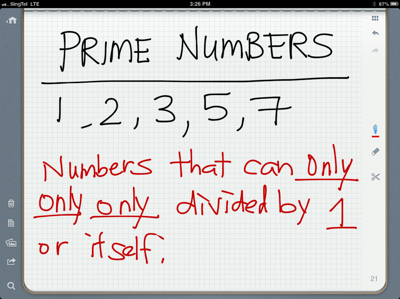So, we advanced ourselves to play the game of finding the PRIME NUMBERS.

He is able to find 11-13-17-19.

And of course we stopped at 20.

How we do it?

By mentally inspect each number from 8 to 20.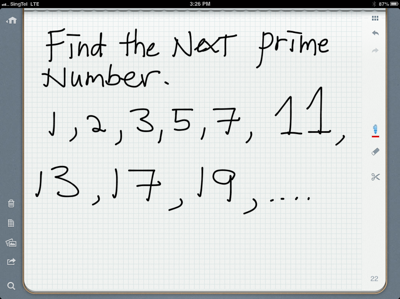While we doing this in mental.

We found something very ODD!

That brings us to Rule #3.

ODD number is ODD ONE OUT.  (The kid loves to use Odd One Out this phrase somehow).

Because, an ODD number can NEVER be divided by an even number.

They have learned odd and even concepts.

So, I ask him from 1-20, run thru all numbers to see if that is true?

Jay:  ya.  It is true.

So, why he has to learn this?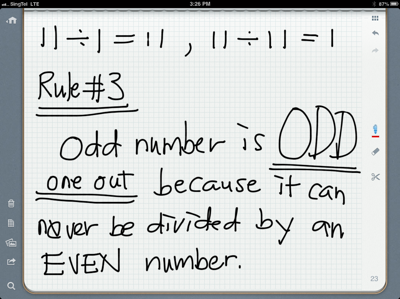Yes!  Evil dad is teaching him SHORT CUTS.

Remember they have to divide the number from 2 onwards?

So, if you are given an ODD number, basically, you can skip the divisions by an even number as Rule #3 tells us that it is impossible.

Kids love Short Cuts.

By using this, the divisions of 11 can skip 5 divisions.  Take out all even numbers.

Jay loves it.

He figured that 11 is another prime number.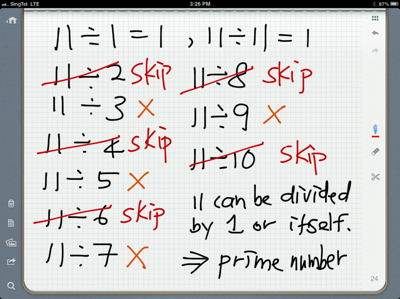One of the familiar way to explain to Jay is by using number bonds.

First set of number bonds being 1 and itself.

Then we proceed to 2 & 6.

Then, 3 & 4.

Then 5, 7, 8, 9, 10, 11 are not possible.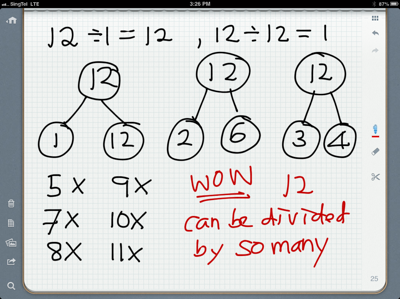To make them understand, you have to let them discover the prime number by themselves.  There, Jay found another prime number.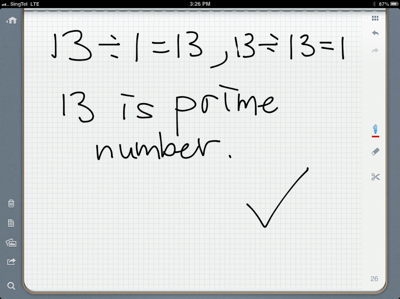It will be not too long that he discover that doing such exploration is so tedious, and very long winded.

There must be a quicker way to do this.

You actually ask them this.  They will say yes yes yes.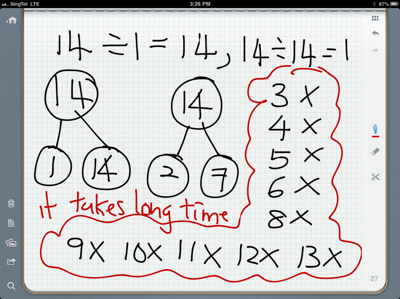So, on the same number bonds, you can conclude one important fact that, after you have successfully divide the number into two prime numbers, it is always impossible to have any numbers to divide.

This is a huge discovery.  And it will become a rule soon.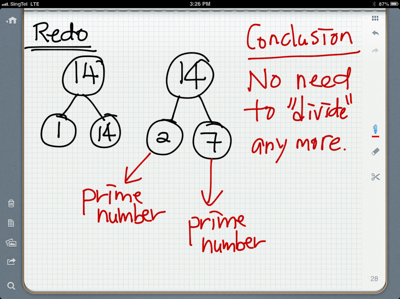Lets strengthen this idea by comparing this 14 to 12.  In the divisions of 12, you notice that 12 can be divided by 1 & 12 (itself).  12 can also be divided by 2 & 6.

Here, you notice that 2 is prime number and 6 is NOT prime number.  So, that means 6 can be furthur divide by 2 & 3.

Sine we found 3, what number is 12 divide by 3?  The answer is 4.

So 12 can be divided by 1, 12, 2, 6, 3 & 4.

Now Jay get a bit confuse.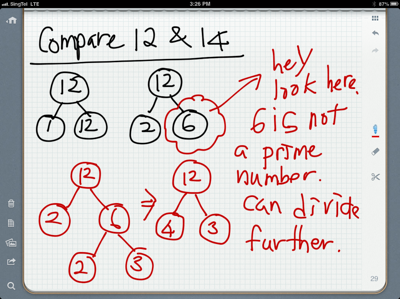The way how we draw multiple number bonds confused Jay.

It is hard to see the link.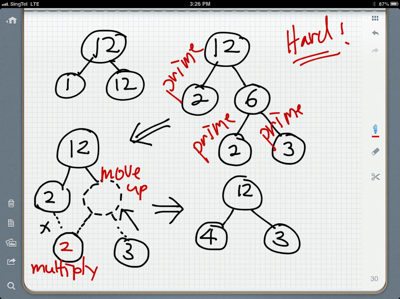It does not matter for now.  Lets move on to 15.  The more example you give the more easier for Jay to see the link.

And now we started to make use of all the rules when number gets bigger and bigger.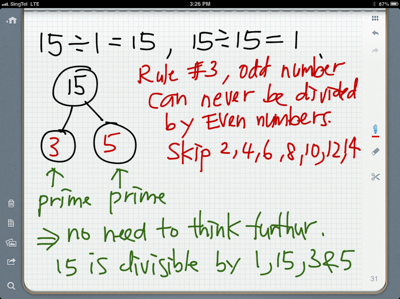And when we come to 16, suddenly I change the way how I drew the number bonds by combining it.
And it works.

Keep divide the non prime nûmber until it become both prime numbers.

And the additional trick is to multiply the left node together.  So in this case, 16 is divisible by 1, 16, 2, 8, and 4.Here Jay Jay finally realize that all even numbers are divisible by 2.

Yeah!

An important observation.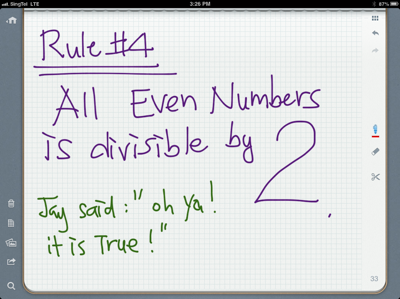We proceed to do 17, and 19.  As both are prime numbers.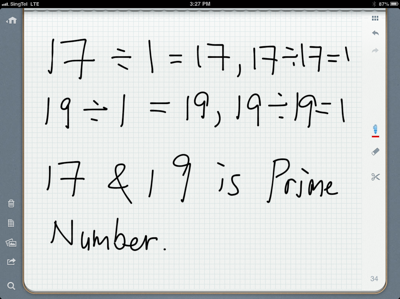Last exercise of the day.  20.And it is a piece of cake.So, that is how I completed the math game of division with Jay Jay, and at the end of the day, he remembers the prime numbers, the 4 rules, and interesting facts about divisions, by discovering them by himself.  Like that hopefully he will learn even more and build the foundation faster,

Oh ya.  Why I decided to do this?

Because... Of this book...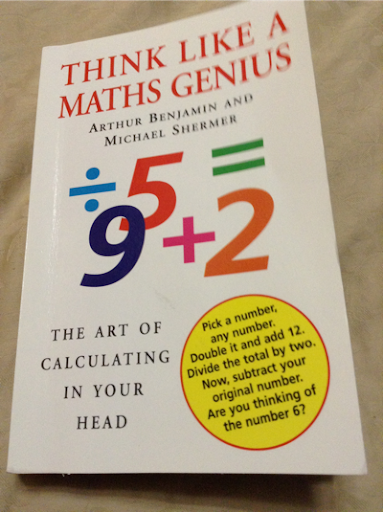And that day, I show him how to multiply 52 x 11 = ?

And put the answer 7 between 52.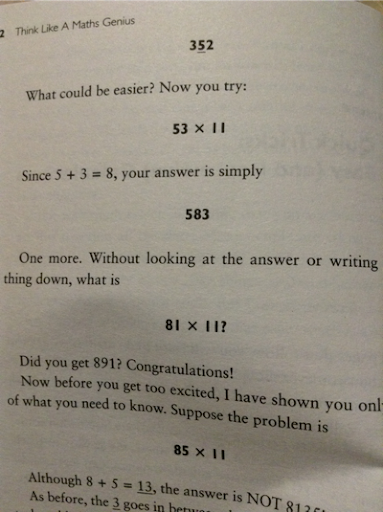Of course 85x11=putting 13 in between 85.  But because 13 is 8+5, and it ends up as double digit, you will have to carry over the tens into the first digit.

So the answer is 935.  Inspired?

Hahaha